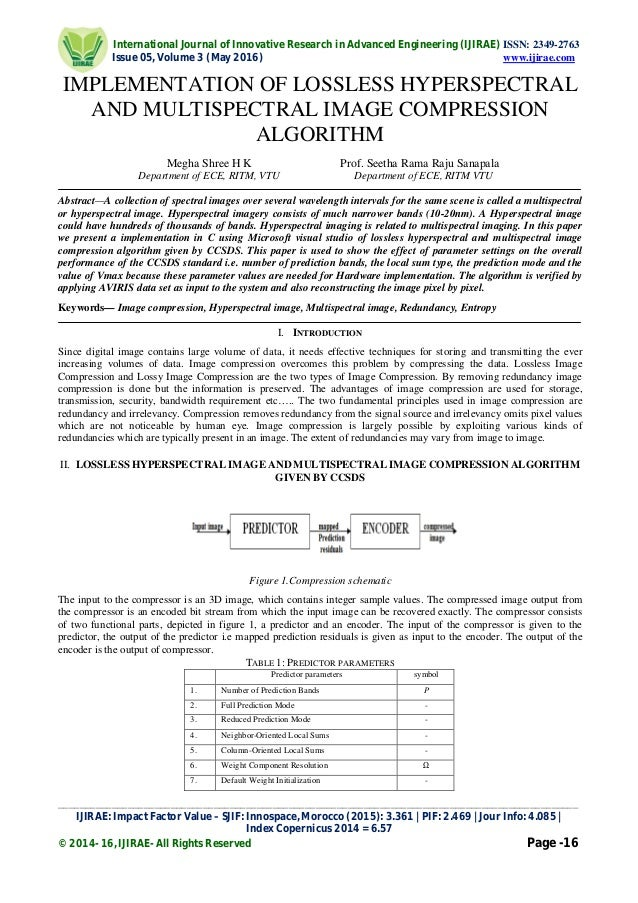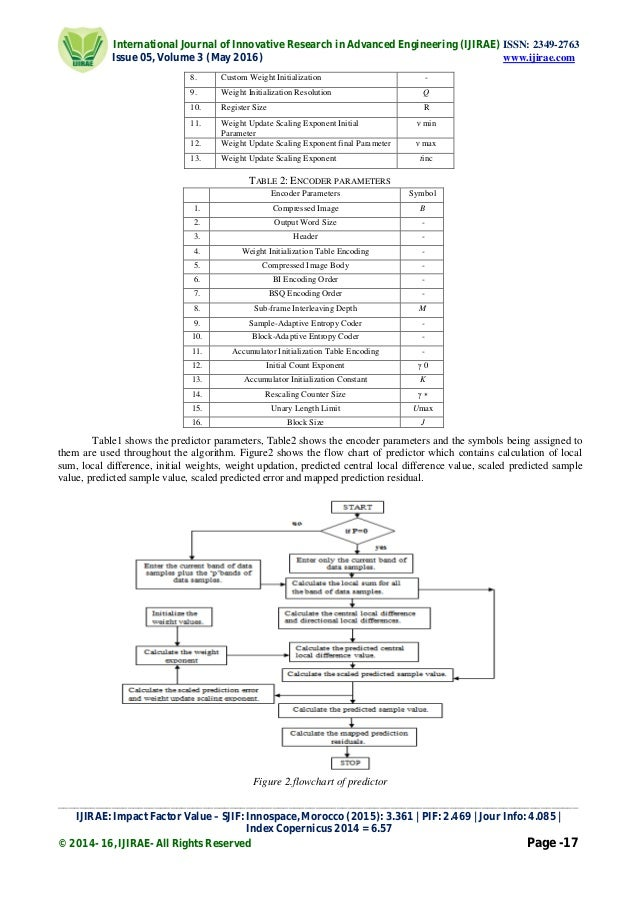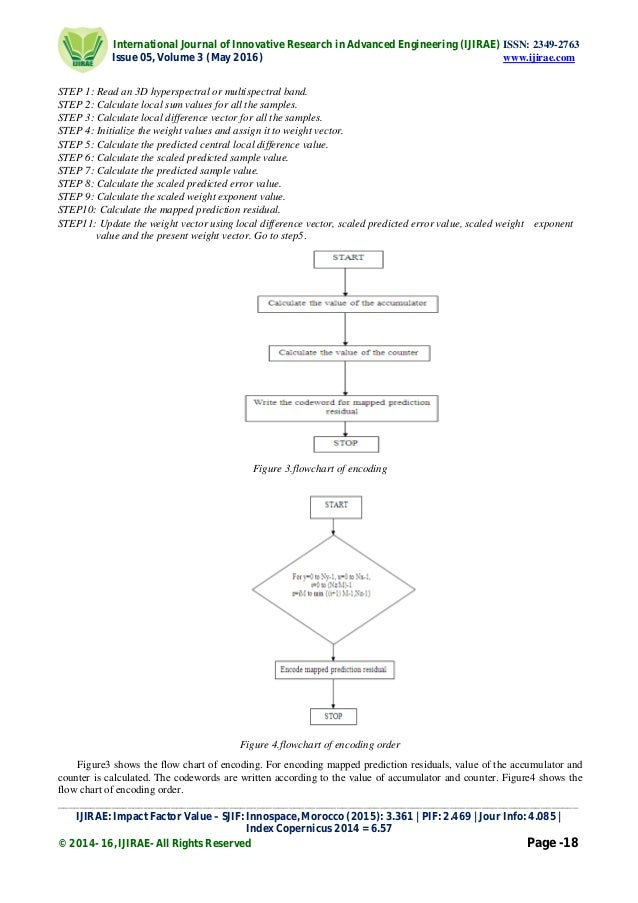Successfully reported this slideshow.Upcoming SlideShare
×

of0

Share

# IMPLEMENTATION OF LOSSLESS HYPERSPECTRAL AND MULTISPECTRAL IMAGE COMPRESSION ALGORITHM

A collection of spectral images over several wavelength intervals for the same scene is called a multispectral or hyperspectral image. Hyperspectral imagery consists of much narrower bands (10-20nm). A Hyperspectral image could have hundreds of thousands of bands. Hyperspectral imaging is related to multispectral imaging. In this paper we present a implementation in C using Microsoft visual studio of lossless hyperspectral and multispectral image compression algorithm given by CCSDS. This paper is used to show the effect of parameter settings on the overall performance of the CCSDS standard i.e. number of prediction bands, the local sum type, the prediction mode and the value of Vmax because these parameter values are needed for Hardware implementation. The algorithm is verified by applying AVIRIS data set as input to the system and also reconstructing the image pixel by pixel.

See all

### Related Audiobooks

#### Free with a 30 day trial from Scribd

See all
• Be the first to like this

### IMPLEMENTATION OF LOSSLESS HYPERSPECTRAL AND MULTISPECTRAL IMAGE COMPRESSION ALGORITHM

1. 1. International Journal of Innovative Research in Advanced Engineering (IJIRAE) ISSN: 2349-2763 Issue 05, Volume 3 (May 2016) www.ijirae.com _________________________________________________________________________________________________ IJIRAE: Impact Factor Value – SJIF: Innospace, Morocco (2015): 3.361 | PIF: 2.469 | Jour Info: 4.085 | Index Copernicus 2014 = 6.57 © 2014- 16, IJIRAE- All Rights Reserved Page -16 IMPLEMENTATION OF LOSSLESS HYPERSPECTRAL AND MULTISPECTRAL IMAGE COMPRESSION ALGORITHM Megha Shree H K Prof. Seetha Rama Raju Sanapala Department of ECE, RITM, VTU Department of ECE, RITM VTU Abstract—A collection of spectral images over several wavelength intervals for the same scene is called a multispectral or hyperspectral image. Hyperspectral imagery consists of much narrower bands (10-20nm). A Hyperspectral image could have hundreds of thousands of bands. Hyperspectral imaging is related to multispectral imaging. In this paper we present a implementation in C using Microsoft visual studio of lossless hyperspectral and multispectral image compression algorithm given by CCSDS. This paper is used to show the effect of parameter settings on the overall performance of the CCSDS standard i.e. number of prediction bands, the local sum type, the prediction mode and the value of Vmax because these parameter values are needed for Hardware implementation. The algorithm is verified by applying AVIRIS data set as input to the system and also reconstructing the image pixel by pixel. Keywords— Image compression, Hyperspectral image, Multispectral image, Redundancy, Entropy I. INTRODUCTION Since digital image contains large volume of data, it needs effective techniques for storing and transmitting the ever increasing volumes of data. Image compression overcomes this problem by compressing the data. Lossless Image Compression and Lossy Image Compression are the two types of Image Compression. By removing redundancy image compression is done but the information is preserved. The advantages of image compression are used for storage, transmission, security, bandwidth requirement etc….. The two fundamental principles used in image compression are redundancy and irrelevancy. Compression removes redundancy from the signal source and irrelevancy omits pixel values which are not noticeable by human eye. Image compression is largely possible by exploiting various kinds of redundancies which are typically present in an image. The extent of redundancies may vary from image to image. II. LOSSLESS HYPERSPECTRAL IMAGE AND MULTISPECTRAL IMAGE COMPRESSION ALGORITHM GIVEN BY CCSDS Figure 1.Compression schematic The input to the compressor is an 3D image, which contains integer sample values. The compressed image output from the compressor is an encoded bit stream from which the input image can be recovered exactly. The compressor consists of two functional parts, depicted in figure 1, a predictor and an encoder. The input of the compressor is given to the predictor, the output of the predictor i.e mapped prediction residuals is given as input to the encoder. The output of the encoder is the output of compressor. TABLE 1: PREDICTOR PARAMETERS Predictor parameters symbol 1. Number of Prediction Bands P 2. Full Prediction Mode - 3. Reduced Prediction Mode - 4. Neighbor-Oriented Local Sums - 5. Column-Oriented Local Sums - 6. Weight Component Resolution Ω 7. Default Weight Initialization -
2. 2. International Journal of Innovative Research in Advanced Engineering (IJIRAE) ISSN: 2349-2763 Issue 05, Volume 3 (May 2016) www.ijirae.com _________________________________________________________________________________________________ IJIRAE: Impact Factor Value – SJIF: Innospace, Morocco (2015): 3.361 | PIF: 2.469 | Jour Info: 4.085 | Index Copernicus 2014 = 6.57 © 2014- 16, IJIRAE- All Rights Reserved Page -17 8. Custom Weight Initialization - 9. Weight Initialization Resolution Q 10. Register Size R 11. Weight Update Scaling Exponent Initial Parameter ν min 12. Weight Update Scaling Exponent final Parameter ν max 13. Weight Update Scaling Exponent tinc TABLE 2: ENCODER PARAMETERS Encoder Parameters Symbol 1. Compressed Image B 2. Output Word Size - 3. Header - 4. Weight Initialization Table Encoding - 5. Compressed Image Body - 6. BI Encoding Order - 7. BSQ Encoding Order - 8. Sub-frame Interleaving Depth M 9. Sample-Adaptive Entropy Coder - 10. Block-Adaptive Entropy Coder - 11. Accumulator Initialization Table Encoding - 12. Initial Count Exponent γ 0 13. Accumulator Initialization Constant K 14. Rescaling Counter Size γ ∗ 15. Unary Length Limit Umax 16. Block Size J Table1 shows the predictor parameters, Table2 shows the encoder parameters and the symbols being assigned to them are used throughout the algorithm. Figure2 shows the flow chart of predictor which contains calculation of local sum, local difference, initial weights, weight updation, predicted central local difference value, scaled predicted sample value, predicted sample value, scaled predicted error and mapped prediction residual. Figure 2.flowchart of predictor
3. 3. International Journal of Innovative Research in Advanced Engineering (IJIRAE) ISSN: 2349-2763 Issue 05, Volume 3 (May 2016) www.ijirae.com _________________________________________________________________________________________________ IJIRAE: Impact Factor Value – SJIF: Innospace, Morocco (2015): 3.361 | PIF: 2.469 | Jour Info: 4.085 | Index Copernicus 2014 = 6.57 © 2014- 16, IJIRAE- All Rights Reserved Page -18 STEP 1: Read an 3D hyperspectral or multispectral band. STEP 2: Calculate local sum values for all the samples. STEP 3: Calculate local difference vector for all the samples. STEP 4: Initialize the weight values and assign it to weight vector. STEP 5: Calculate the predicted central local difference value. STEP 6: Calculate the scaled predicted sample value. STEP 7: Calculate the predicted sample value. STEP 8: Calculate the scaled predicted error value. STEP 9: Calculate the scaled weight exponent value. STEP10: Calculate the mapped prediction residual. STEP11: Update the weight vector using local difference vector, scaled predicted error value, scaled weight exponent value and the present weight vector. Go to step5. Figure 3.flowchart of encoding Figure 4.flowchart of encoding order Figure3 shows the flow chart of encoding. For encoding mapped prediction residuals, value of the accumulator and counter is calculated. The codewords are written according to the value of accumulator and counter. Figure4 shows the flow chart of encoding order.
4. 4. International Journal of Innovative Research in Advanced Engineering (IJIRAE) ISSN: 2349-2763 Issue 05, Volume 3 (May 2016) www.ijirae.com _________________________________________________________________________________________________ IJIRAE: Impact Factor Value – SJIF: Innospace, Morocco (2015): 3.361 | PIF: 2.469 | Jour Info: 4.085 | Index Copernicus 2014 = 6.57 © 2014- 16, IJIRAE- All Rights Reserved Page -19 Under band interleaving encoding order, samples in an image will be encoded in the order defined by the nesting of sample index loops. Sample input taken for analysis is AVIRISSC10 Hyperspectral image and the specifications are mentioned below. III. IMPLEMENTATION RESULTS IN C USING VISUAL STUDIO Figure5: Varying number of prediction bands with full column mode. Figure6: Varying number of prediction bands with full neighbour mode. Figure7: Varying number of prediction bands with reduced column mode.
5. 5. International Journal of Innovative Research in Advanced Engineering (IJIRAE) ISSN: 2349-2763 Issue 05, Volume 3 (May 2016) www.ijirae.com _________________________________________________________________________________________________ IJIRAE: Impact Factor Value – SJIF: Innospace, Morocco (2015): 3.361 | PIF: 2.469 | Jour Info: 4.085 | Index Copernicus 2014 = 6.57 © 2014- 16, IJIRAE- All Rights Reserved Page -20 Figure 8: Varying number of prediction bands with reduced neighbour mode. Figure 9: Varying vmax, keeping tinc=16 and vmin=-1 Figure 10: Varying vmin and keeping vmax=9 Figure 11: Varying Ω and keeping R=64 Figure 12: Varying R and keeping Ω=16
6. 6. International Journal of Innovative Research in Advanced Engineering (IJIRAE) ISSN: 2349-2763 Issue 05, Volume 3 (May 2016) www.ijirae.com _________________________________________________________________________________________________ IJIRAE: Impact Factor Value – SJIF: Innospace, Morocco (2015): 3.361 | PIF: 2.469 | Jour Info: 4.085 | Index Copernicus 2014 = 6.57 © 2014- 16, IJIRAE- All Rights Reserved Page -21 Figure 13: Varying γ*and keeping γ0=1 Figure 14: Varying block size with P=15 Figure 15: Encoding with block full neighbor mode. Figure 16: Varying k, keeping γ*=4 and γ0=3 Figure 17: Varying k, keeping γ*=8 and γ0=3
7. 7. International Journal of Innovative Research in Advanced Engineering (IJIRAE) ISSN: 2349-2763 Issue 05, Volume 3 (May 2016) www.ijirae.com _________________________________________________________________________________________________ IJIRAE: Impact Factor Value – SJIF: Innospace, Morocco (2015): 3.361 | PIF: 2.469 | Jour Info: 4.085 | Index Copernicus 2014 = 6.57 © 2014- 16, IJIRAE- All Rights Reserved Page -22 Figure 18: Varying k, keeping γ*=6 and γ0=1 Varying number of prediction bands with full column mode, full neighbor mode, reduced column mode and reduced neighbor mode are shown in figure 5, figure 6, figure 7 and figure 8. It can be observed in the graph that without prediction bands the bit rate is higher, when the number of prediction bands increases then according the bit rate will change shown in figure 5, figure 6, figure 7 and figure 8. Figure 9 shows the graph plotted by varying vmax (keeping vmin=-1). Figure 10 shows the graph plotted by varying vmin (keeping vmax=9). Figure 11 shows the graph plotted by varying Ω(keeping R=64). Figure 12 shows the graph plotted by varying R (keeping Ω=64). Figure 13 shows the graph plotted by varying γ*(keeping γ0=1). Figure 14 shows the graph plotted by varying block size(keeping P=15). Figure 15 shows Encoding with block full neighbor mode. Figure 16 shows the graph plotted by Varying k( keeping γ*=4 and γ0=3). Figure 17 shows the graph plotted by Varying k( keeping γ*=8 and γ0=3). Figure 18 shows the graph plotted by Varying k( keeping γ*=6 and γ0=1). All the graphs are plotted by varying predictor and encoder parameters versus bit rate of the compressed image. In all the graphs from figure5 to figure18, y-axis represents the compressed bit rate in samples/sec. Column-oriented local sums combined with reduced mode gives the best performance when compared with full neighbor mode, full column mode and reduced neighbor mode. From the analysis done it can be seen that the predictor parameters tinc and vmin have little effect on compression performance, and vmax is the most important parameter. As vmax increases, compressed bit rate also increases gradually. The choice of compression parameters affects not only compression performance but also complexity. From figure 11 and figure 12 it is seen that Ω has a noticeable effect on the compression performance, while R does not. Figure 13 suggests that the effect of these parameters on compressed data rate performance is absolutely marginal. It is also shown in the figure 16, figure 17 and figure 18 that compression ratio does not seem to depend much on K. IV.CONCLUSION Lossless hyperspectral and multispectral image compression algorithm given by CCSDS implementation was done successfully in C using visual studio. This paper shows the effect of parameter settings on the overall performance of the CCSDS standard i.e. number of previous bands, the local sum type, the value of Vmax, the value of Vmin, the value of tinc and so on. The computational complexity depends on the number of prediction bands P, the local sum type and the prediction mode, as the number of previous bands increases the computational complexity also increases. It seems appropriate to use column oriented and reduced prediction modes. The algorithm is verified by applying AVIRIS data set as input and reconstruction of the image is done. Compression ratio of nearly 3 is obtained for P>=0. Compression system is used to reduce higher memory requirement. REFERENCES . Lossless hyperspectral and multispectral image Compression CCSDS Blue Book. . “Lossless Compression of Multi/Hyper-SpectralImagery Based on a 3-D Fuzzy Prediction “,IEEE TRANSACTIONS ON GEOSCIENCE AND REMOTE SENSING, VOL. 37, NO. 5, SEPTEMBER 1999 2287. . ”Methodand System for Compression of Hyperspectral or Multispectral imagery with a Global Optimal Compression Algorithm” (GOCA) US 20110007819 A1 . ”Kronecker-Product Gain-Shape Vector Quantization for Multispectral and Hyperspectral . Image Coding”, IEEE TRANSACTIONS ON IMAGE PROCESSING, VOL. 7, NO. 5, MAY 1998. . ”FPGA Implementation of the CCSDS-123 B-1 LooslessHyperspectral Image Compression Algorithm Prediction Stage” by Giorgio Lopez, Ettore Napoli, Antoni G.M. Strollo,university of napoli. . ”Analysis of Hyperspectral and Compression algorithm pararmeters” by ShwethaKarnik S and A.R. Aswatha, department of telecommunication, dayanandsagar College of Engineering, Bangalore. . ”Hardware Implementation of local sum and Difference Module for hyperspectral image Compression”by ShwethaKarnik S and A.R. Aswatha, department of telecommunication, dayanandsagar College of Engineering, Bangalore.

Total views

95

On Slideshare

0

From embeds

0

Number of embeds

1

2

Shares

0